# 06-240/Classnotes For Tuesday November 21

## More about the Wongpak Matrices

In Talk:06-240/Classnotes_For_Tuesday_November_14, User:Wongpak asked something about row echelon form and reduced row echelon form, and gave the following matrices as specific examples: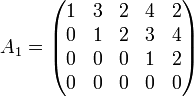$A_1=\begin{pmatrix}1&3&2&4&2\\0&1&2&3&4\\0&0&0&1&2\\0&0&0&0&0 \end{pmatrix}$$A_2=\begin{pmatrix}1&0&-4&0&-6\\0&1&2&0&-2\\0&0&0&1&2\\0&0&0&0&0 \end{pmatrix}$

So let us assume row reduction leads us to the systems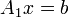$A_1x=b$ or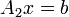$A_2x=b$. What does it tell us about the solutions? Let us start from the second system: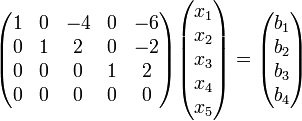$\begin{pmatrix}1&0&-4&0&-6\\0&1&2&0&-2\\0&0&0&1&2\\0&0&0&0&0\end{pmatrix} \begin{pmatrix}x_1\\x_2\\x_3\\x_4\\x_5\end{pmatrix} = \begin{pmatrix}b_1\\b_2\\b_3\\b_4\end{pmatrix}$ or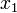$x_1$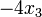$-4x_3$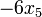$-6x_5$$=$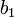$b_1$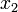$x_2$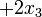$+2x_3$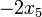$-2x_5$$=$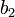$b_2$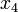$x_4$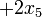$+2x_5$$=$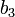$b_3$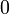$0$$=$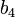$b_4$

Well, quite clearly if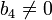$b_4\neq 0$ this system has no solutions, but if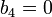$b_4=0$ it has solutions no matter what$b_1$,$b_2$ and$b_3$ are. Finally, for any given values of$b_1$,$b_2$ and$b_3$ we can choose the values of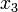$x_3$ and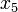$x_5$ (the variables corresponding the columns containing no pivots) as we please, and then get solutions by setting the "pivotal variables" in terms of the non-pivotal ones as follows: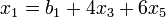$x_1=b_1+4x_3+6x_5$,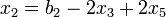$x_2=b_2-2x_3+2x_5$ and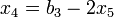$x_4=b_3-2x_5$.

What about the system corresponding to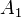$A_1$? It is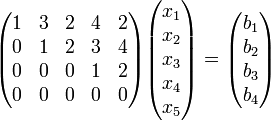$\begin{pmatrix}1&3&2&4&2\\0&1&2&3&4\\0&0&0&1&2\\0&0&0&0&0\end{pmatrix} \begin{pmatrix}x_1\\x_2\\x_3\\x_4\\x_5\end{pmatrix} = \begin{pmatrix}b_1\\b_2\\b_3\\b_4\end{pmatrix}$ or$x_1$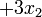$+3x_2$$+2x_3$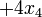$+4x_4$$+2x_5$$=$$b_1$$x_2$$+2x_3$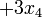$+3x_4$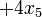$+4x_5$$=$$b_2$$x_4$$+2x_5$$=$$b_3$$0$$=$$b_4$

Here too we have solutions iff$b_4=0$, and if$b_4=0$, we have the freedom to choose the non-pivotal variables$x_3$ and$x_5$ as we please. But now the formulas for fixing the pivotal variables$x_1$,$x_2$ and$x_4$ in terms of the non-pivotal ones are a bit harder.